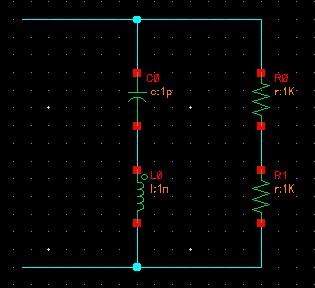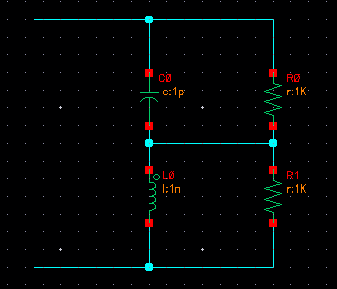# input impedance of RLC tank, and characteristic impedance

Status
Not open for further replies.

#### dxt78

##### Newbie level 4
Hello,
I have an RLC tank as shown in attachment (2R in parallel with an L and a C). I have two questions about it:
1) Why is the characteristic impedance of this LC tank sqrt(L/C) and what meaning does it have?
2) When computing the input resistance of this tank, what role should the characteristic impedance play?
Thanks.There is one single frequency wo=1/sqrt(LC) for which the reactive conductance of the capacitor
and of the inductor (wC and 1/wL), respectively, are equal.

If woC=1/woL you can insert the above expression for wo and you get:
woC=sqrt(C/L) and woL=sqrt(L/C).

In words: The characteristic impedance is the impedance of both reactive elements for w=wo (resonant frequency).

Last edited:

That means, at w=wo the conductance of the whole circuit is real and equals Y=1/R.
For the present circuit (ideal L and C), Y would be inifinite.

•LvW

### LvW

Points: 2
Thanks
LvW

LvW, thank you so much for your detailed reply. I suppose I was getting caught up in the definition of characteristic impedance as it pertains to transmission lines, but the math that you have described makes perfect sense. I am actually pursuing this problem as a way to calculate the input impedance of the circuit attached here:Using what you've described, I calculate Rin as 2R/[1+R/Z0], although...I have also seen it as 2R/[1+(R/Z0)^2]?? I must be making a mistake somewhere in the calculation.

---------- Post added at 18:36 ---------- Previous post was at 17:43 ----------

LvW, thank you so much for your detailed reply. I suppose I was getting caught up in the definition of characteristic impedance as it pertains to transmission lines, but the math that you have described makes perfect sense. I am actually pursuing this problem as a way to calculate the input impedance of the circuit attached here:Using what you've described, I calculate Rin as 2R/[1+R/Z0], although...I have also seen it as 2R/[1+(R/Z0)^2]?? I must be making a mistake somewhere in the calculation.

Actually the derivation described above is all wrong. If you account for the +j and -j for the inductor and capacitor impedances, you will get 2R/[1+(R/Z0)^2]

Status
Not open for further replies.